# Area of Composite Figures Lesson Plan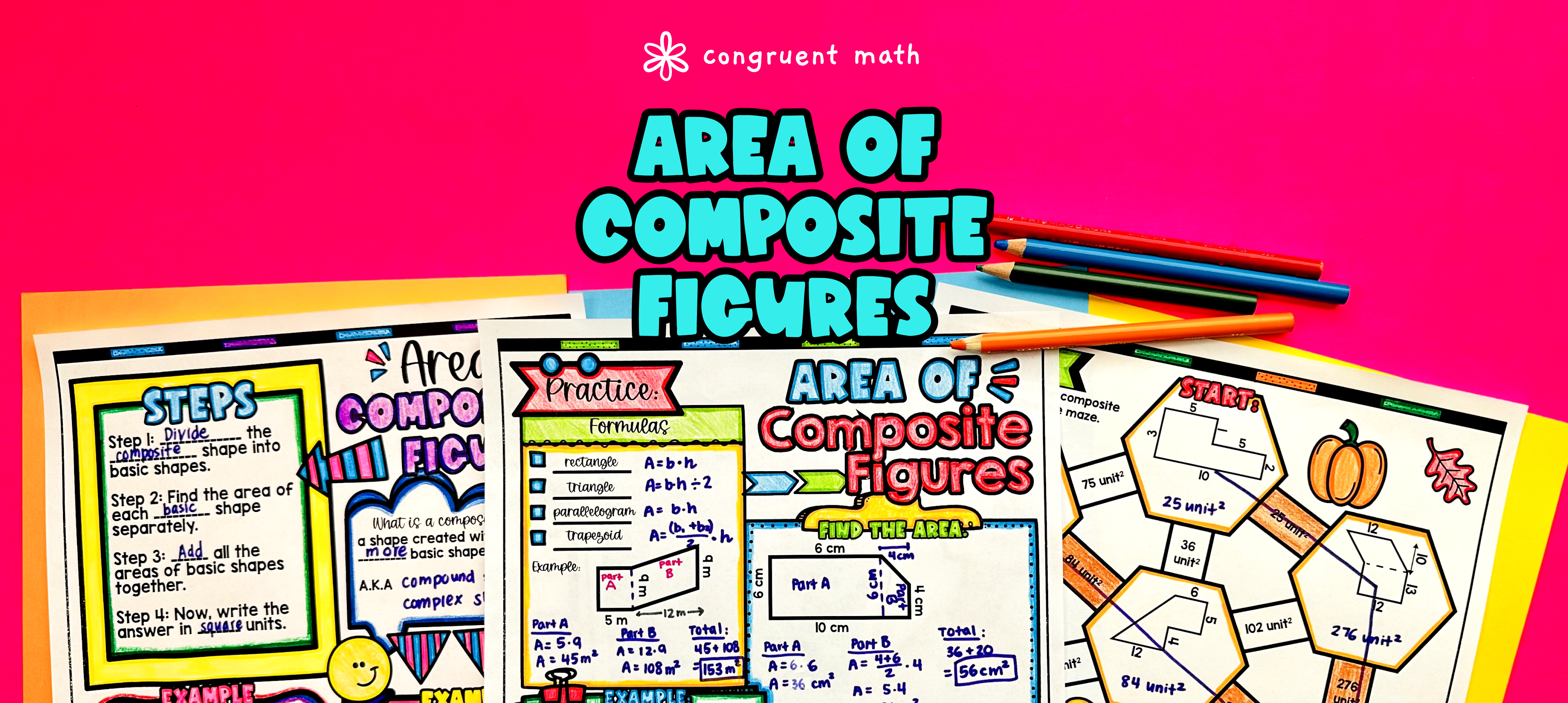Pin This

## Overview

Ever wondered how to teach the area of composite figures in an engaging way to your sixth and seventh grade students?

In this lesson plan, students will learn about finding the area of composite figures made up of rectangles, trapezoids, parallelograms, and triangles. Through artistic and interactive guided notes, check for understanding activities, a practice coloring worksheet, and a maze worksheet, students will gain a comprehensive understanding of this topic.

The lesson culminates with a real-life application activity where students will read and write about the real-life uses of the area of composite figures. This activity helps students make connections between the math they are learning and its practical applications in the real world.

## Get the Lesson Materials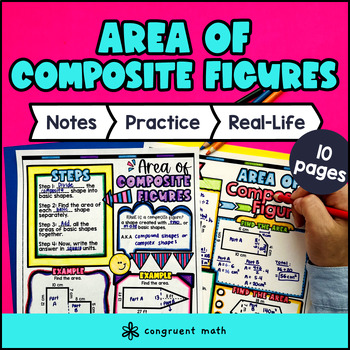\$4.25

## Learning Objectives

After this lesson, students will be able to:

• Calculate the area of composite figures by decomposing them into basic polygons

• Divide up and calculate the area of different types of polygons (rectangles, trapezoids, parallelograms, and triangles) and then sum them up to find area of a composite figure

• Apply the concept of area of composite figures to real-life situations and explain their significance

## Prerequisites

Before this lesson, students should be familiar with:

• basic operations with whole numbers and decimals

• know how to substitute a number into a variable

## Key Vocabulary

• Composite figures

• Rectangles

• Trapezoids

• Parallelograms

• Triangles

• Decomposing

• Basic polygons

## Procedure

### Introduction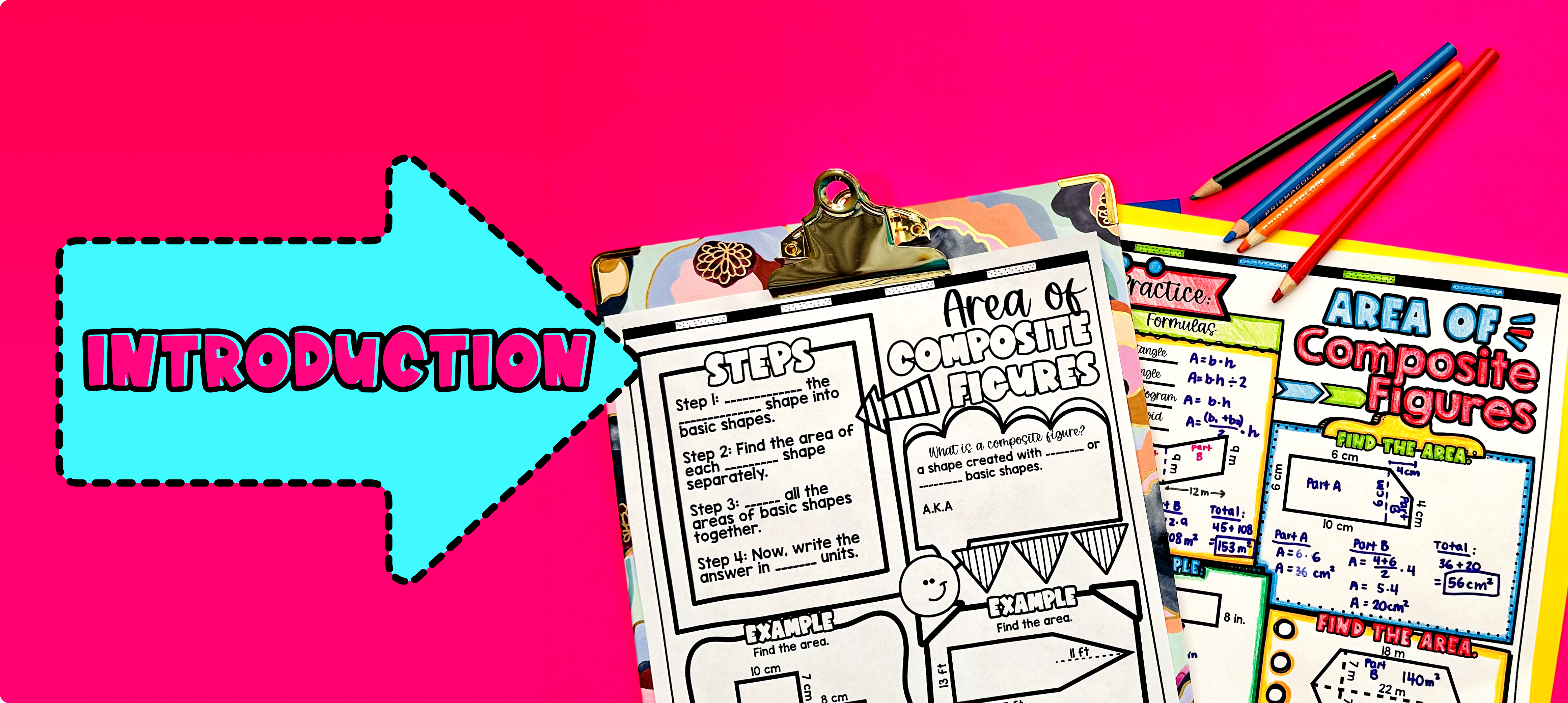Pin This

As a hook, ask students why understanding the concept of area of composite figures is important in real life. You can provide an example such as, "Think about a garden that has a rectangular lawn and a triangular flower bed. How would you calculate the total area of the garden?" Use the last page of the guided notes as well as the FAQs below for additional ideas and examples related to real-life applications of area of composite figures.

Use the guided notes to introduce the topic of area of composite figures and how to calculate it by decomposing into basic polygons. Begin by explaining that composite figures are made up of multiple polygons, such as rectangles, trapezoids, parallelograms, and triangles. Emphasize that to find the total area of a composite figure, we need to find the individual areas of each component shape and then add them together.

Walk through the key points of the topic covered in the first two pages of the guided notes, such as:

1. Decomposing a composite figure into basic polygons.

2. Calculating the area of each individual shape using the appropriate formulas.

3. Adding the areas of all the individual shapes to find the total area of the composite figure.

Refer to the FAQ section below the guided notes for a walk through on how to teach these key points and for ideas on how to respond to common student questions.

Based on student responses and understanding, reteach any concepts that students may need extra help with. If your class has a wide range of proficiency levels, you can pull out students for reteaching while more advanced students begin working on the practice exercises.

### Practice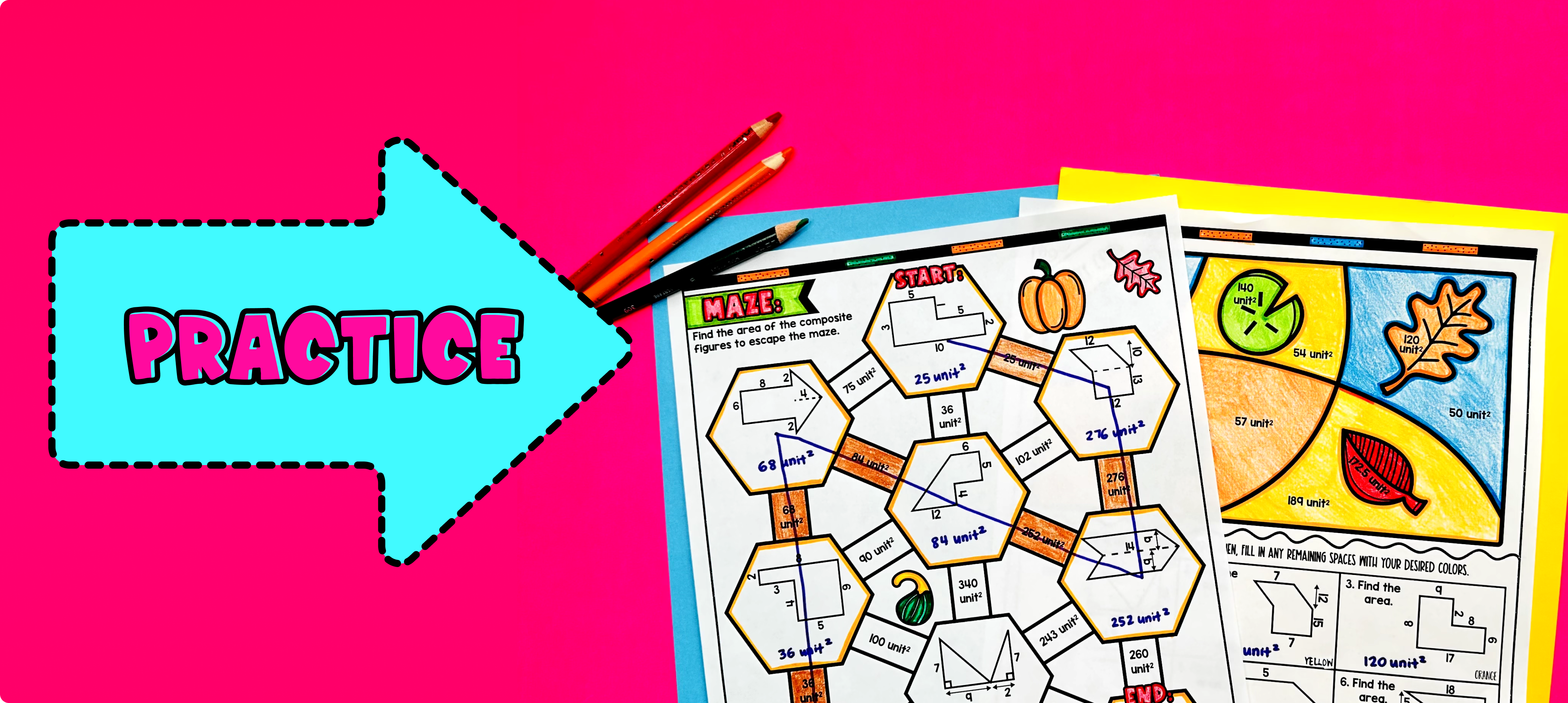Pin This

Have students practice calculating the area of composite figures by using the practice worksheets provided in the resource. Walk around the classroom to answer any questions that students may have while they work on the worksheets.

Fast finishers can dive into the maze activity and color by number activity included in the resource for extra practice. You can assign it as homework for the remainder of the class.

### Real-Life Application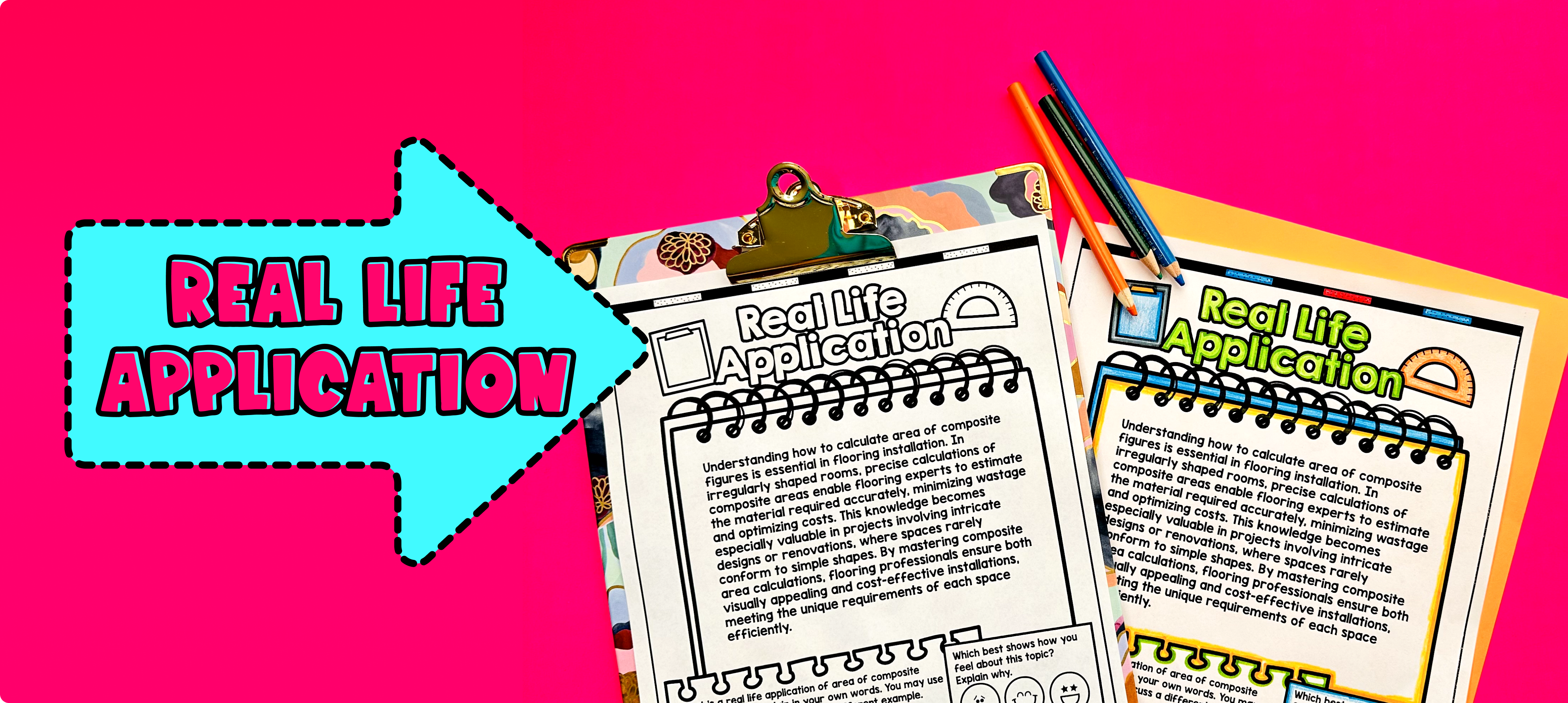Pin This

Use the last page of the guided notes, "real life applications", to bring the class back together, and introduce the concept of real-life applications of the area of composite figures. Discuss how the area of composite figures is used in real-world situations to solve practical problems.

Refer to the FAQ section of the teaching resource for more ideas and examples on how to teach the real-life applications of the area of composite figures. This section provides additional resources and suggestions to help you engage and motivate your students with real-world connections.

## Extensions

If you’re looking for digital practice for area of composite figures, try my Pixel Art activities in Google Sheets. Every answer is automatically checked, and correct answers unlock parts of a mystery picture. It’s incredibly fun, and a powerful tool for differentiation.

Here is one activity to explore: